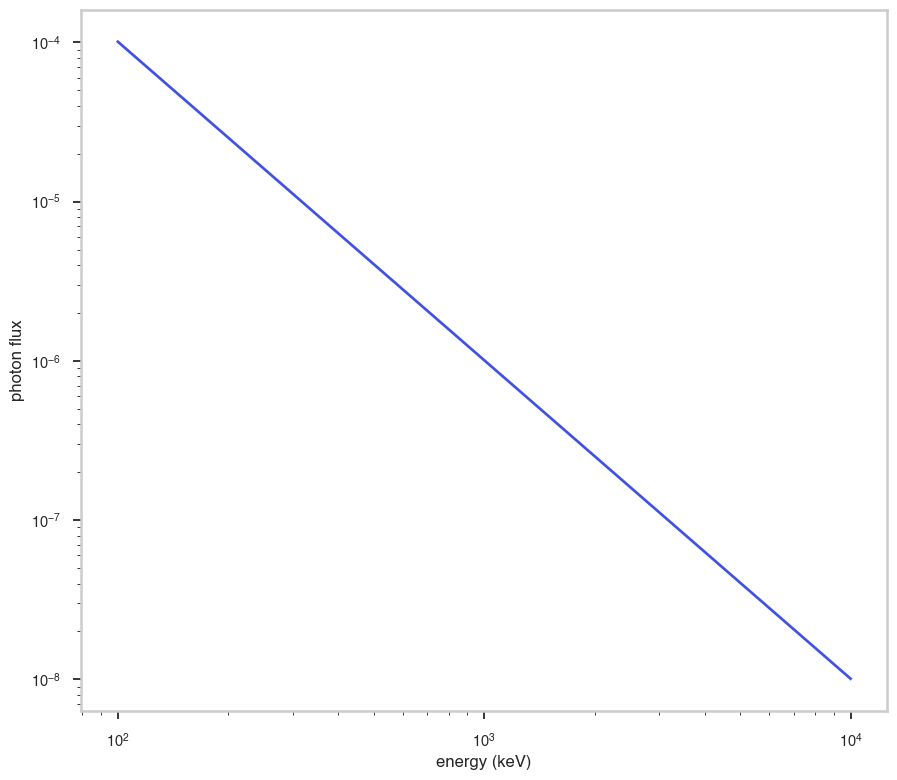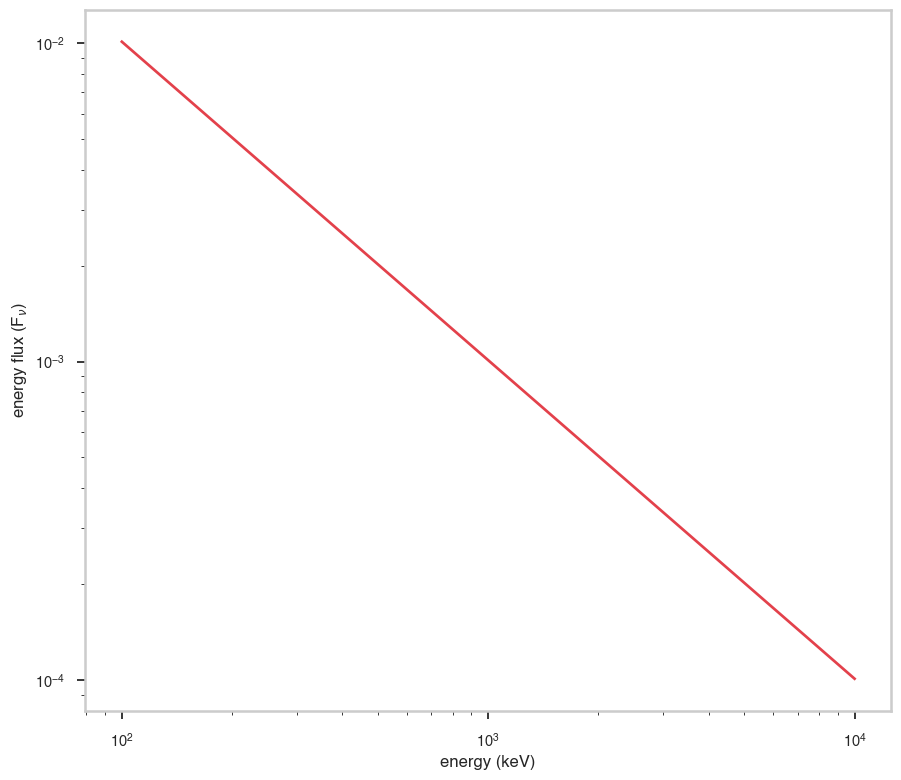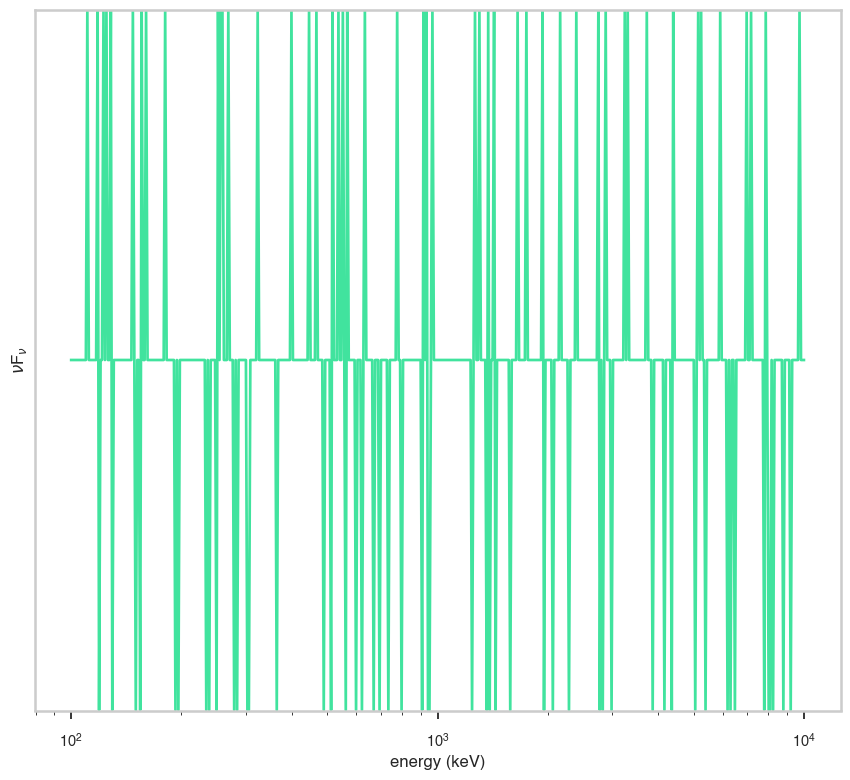# Powerlaw flux

:

# Parameters
func_name = "Powerlaw_flux"
wide_energy_range = True
x_scale = "log"
y_scale = "log"
linear_range = False


## Description

:

func.display()

• description: A simple power-law with the photon flux in a band used as normalization. This will reduce the correlation between the index and the normalization.
• formula: $\frac{F(\gamma+1)} {b^{\gamma+1} - a^{\gamma+1}} (x)^{\gamma}$
• parameters:
• F:
• value: 1.0
• desc: Integral between a and b
• min_value: 1e-30
• max_value: 1000.0
• unit:
• is_normalization: True
• delta: 0.1
• free: True
• index:
• value: -2.0
• desc: Photon index
• min_value: -10.0
• max_value: 10.0
• unit:
• is_normalization: False
• delta: 0.2
• free: True
• a:
• value: 1.0
• desc: lower bound for the band in which computing the integral F
• min_value: None
• max_value: None
• unit:
• is_normalization: False
• delta: 0.1
• free: False
• b:
• value: 100.0
• desc: upper bound for the band in which computing the integral F
• min_value: None
• max_value: None
• unit:
• is_normalization: False
• delta: 10.0
• free: False

## Shape

The shape of the function.

If this is not a photon model but a prior or linear function then ignore the units as these docs are auto-generated

:

fig, ax = plt.subplots()

ax.plot(energy_grid, func(energy_grid), color=blue)

ax.set_xlabel("energy (keV)")
ax.set_ylabel("photon flux")
ax.set_xscale(x_scale)
ax.set_yscale(y_scale)## F$$_{\nu}$$

The F$$_{\nu}$$ shape of the photon model if this is not a photon model, please ignore this auto-generated plot

:

fig, ax = plt.subplots()

ax.plot(energy_grid, energy_grid * func(energy_grid), red)

ax.set_xlabel("energy (keV)")
ax.set_ylabel(r"energy flux (F$_{\nu}$)")
ax.set_xscale(x_scale)
ax.set_yscale(y_scale)## $$\nu$$F$$_{\nu}$$

The $$\nu$$F$$_{\nu}$$ shape of the photon model if this is not a photon model, please ignore this auto-generated plot

:

fig, ax = plt.subplots()

ax.plot(energy_grid, energy_grid**2 * func(energy_grid), color=green)

ax.set_xlabel("energy (keV)")
ax.set_ylabel(r"$\nu$F$_{\nu}$")
ax.set_xscale(x_scale)
ax.set_yscale(y_scale)